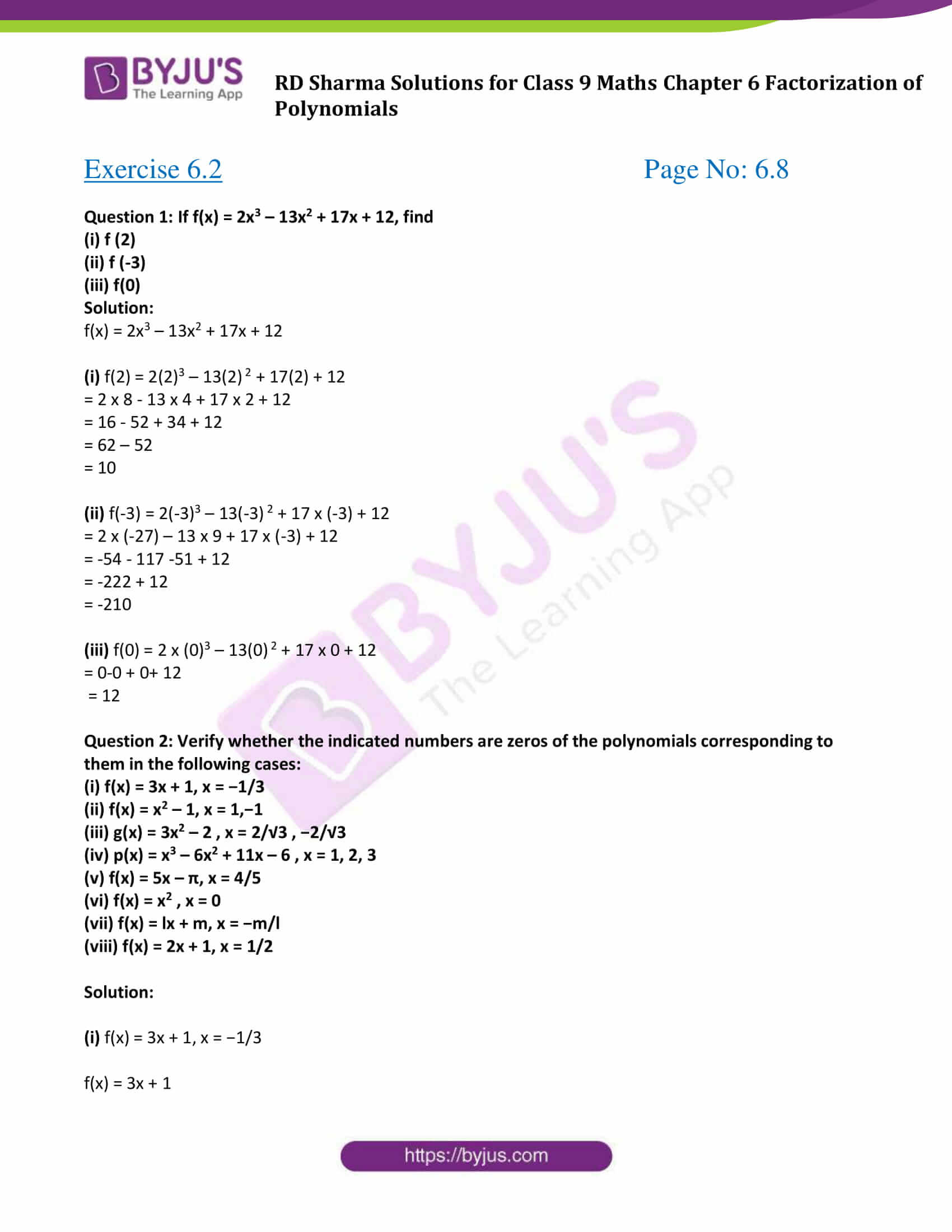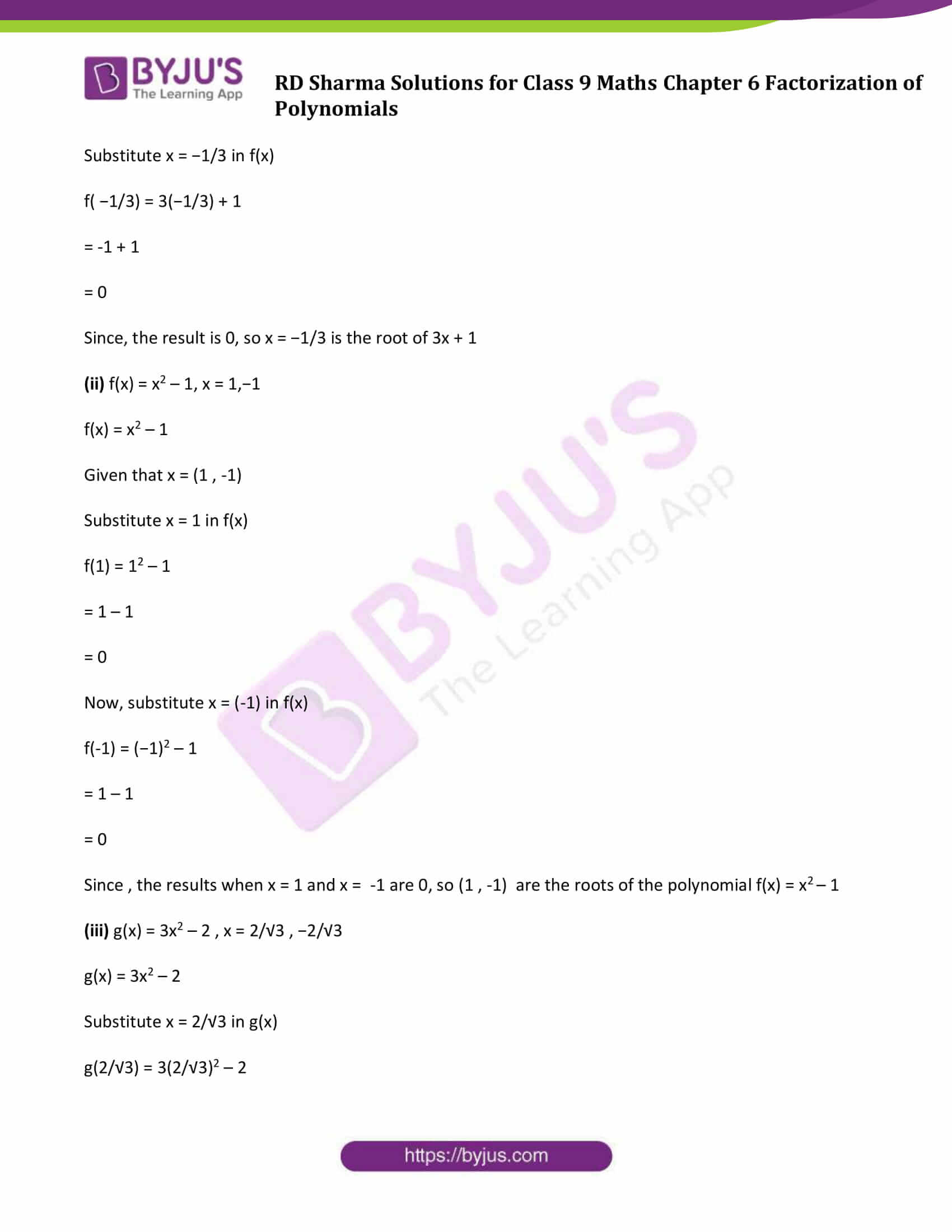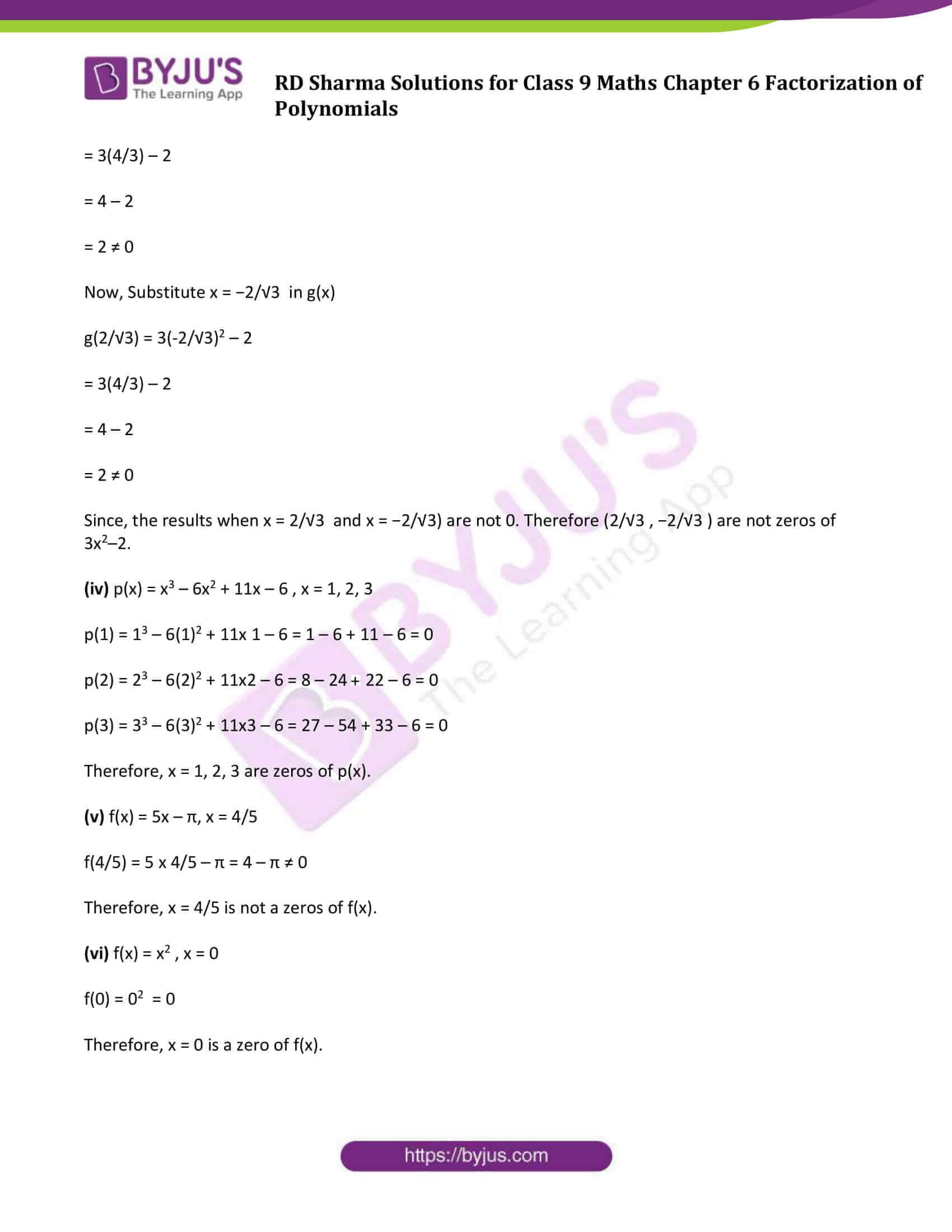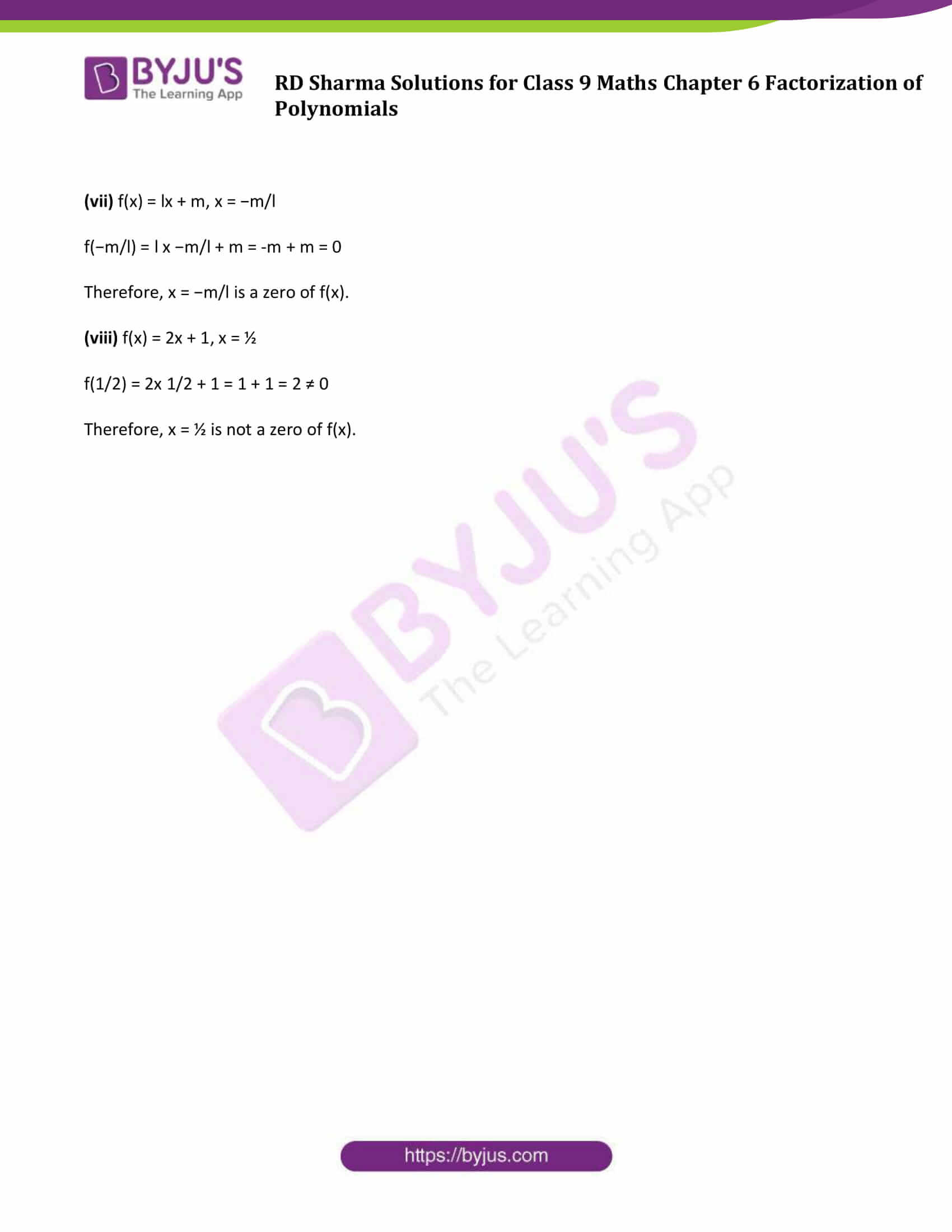# RD Sharma Solutions for Class 9 Maths Chapter 6 Factorization of Polynomials Exercise 6.2

RD Sharma Class 9 Mathematics Chapter 6 Exercise 6.2 Factorization of Polynomials is provided here. In this exercise, students will learn how to find the value of a polynomial. This study material helps students to master a concept by solving various questions enlisted in this exercise. For more details, students can practice RD Sharma Class 9 chapter 6 solutions.

## Download PDF of RD Sharma Solutions for Class 9 Maths Chapter 6 Factorization of Polynomials Exercise 6.2### Access Answers to Maths RD Sharma Solutions for Class 9 Chapter 6 Factorization of Polynomials Exercise 6.2 Page number 6.8

#### Exercise 6.2 Page No: 6.8

Question 1: If f(x) = 2x3 – 13x2 + 17x + 12, find

(i) f (2)

(ii) f (-3)

(iii) f(0)

Solution:

f(x) = 2x3 – 13x2 + 17x + 12

(i) f(2) = 2(2)3 – 13(2) 2 + 17(2) + 12

= 2 x 8 – 13 x 4 + 17 x 2 + 12

= 16 – 52 + 34 + 12

= 62 – 52

= 10

(ii) f(-3) = 2(-3)3 – 13(-3) 2 + 17 x (-3) + 12

= 2 x (-27) – 13 x 9 + 17 x (-3) + 12

= -54 – 117 -51 + 12

= -222 + 12

= -210

(iii) f(0) = 2 x (0)3 – 13(0) 2 + 17 x 0 + 12

= 0-0 + 0+ 12

= 12

Question 2: Verify whether the indicated numbers are zeros of the polynomials corresponding to them in the following cases:

(i) f(x) = 3x + 1, x = −1/3

(ii) f(x) = x2 – 1, x = 1,−1

(iii) g(x) = 3x2 – 2 , x = 2/√3 , −2/√3

(iv) p(x) = x3 – 6x2 + 11x – 6 , x = 1, 2, 3

(v) f(x) = 5x – π, x = 4/5

(vi) f(x) = x2 , x = 0

(vii) f(x) = lx + m, x = −m/l

(viii) f(x) = 2x + 1, x = 1/2

Solution:

(i) f(x) = 3x + 1, x = −1/3

f(x) = 3x + 1

Substitute x = −1/3 in f(x)

f( −1/3) = 3(−1/3) + 1

= -1 + 1

= 0

Since, the result is 0, so x = −1/3 is the root of 3x + 1

(ii) f(x) = x2 – 1, x = 1,−1

f(x) = x2 – 1

Given that x = (1 , -1)

Substitute x = 1 in f(x)

f(1) = 12 – 1

= 1 – 1

= 0

Now, substitute x = (-1) in f(x)

f(-1) = (−1)2 – 1

= 1 – 1

= 0

Since , the results when x = 1 and x = -1 are 0, so (1 , -1) are the roots of the polynomial f(x) = x2 – 1

(iii) g(x) = 3x2 – 2 , x = 2/√3 , −2/√3

g(x) = 3x2 – 2

Substitute x = 2/√3 in g(x)

g(2/√3) = 3(2/√3)2 – 2

= 3(4/3) – 2

= 4 – 2

= 2 ≠ 0

Now, Substitute x = −2/√3 in g(x)

g(2/√3) = 3(-2/√3)2 – 2

= 3(4/3) – 2

= 4 – 2

= 2 ≠ 0

Since, the results when x = 2/√3 and x = −2/√3) are not 0. Therefore (2/√3 , −2/√3 ) are not zeros of 3x2–2.

(iv) p(x) = x3 – 6x2 + 11x – 6 , x = 1, 2, 3

p(1) = 13 – 6(1)2 + 11x 1 – 6 = 1 – 6 + 11 – 6 = 0

p(2) = 23 – 6(2)2 + 11×2 – 6 = 8 – 24 + 22 – 6 = 0

p(3) = 33 – 6(3)2 + 11×3 – 6 = 27 – 54 + 33 – 6 = 0

Therefore, x = 1, 2, 3 are zeros of p(x).

(v) f(x) = 5x – π, x = 4/5

f(4/5) = 5 x 4/5 – π = 4 – π ≠ 0

Therefore, x = 4/5 is not a zeros of f(x).

(vi) f(x) = x2 , x = 0

f(0) = 02 = 0

Therefore, x = 0 is a zero of f(x).

(vii) f(x) = lx + m, x = −m/l

f(−m/l) = l x −m/l + m = -m + m = 0

Therefore, x = −m/l is a zero of f(x).

(viii) f(x) = 2x + 1, x = ½

f(1/2) = 2x 1/2 + 1 = 1 + 1 = 2 ≠ 0

Therefore, x = ½ is not a zero of f(x).

## RD Sharma Solutions for Class 9 Maths Chapter 6 Factorization of Polynomials Exercise 6.2

RD Sharma Solutions Class 9 Maths Chapter 6 Factorization of Polynomials Exercise 6.2 is based on the following topics and subtopics:

• Zeros (roots) of a polynomial
• Value of a polynomial: The value of a polynomial f(x) at x = a is obtained by substituting x = a in the given polynomial and is denoted by f(a).
• Results of some Important theorem